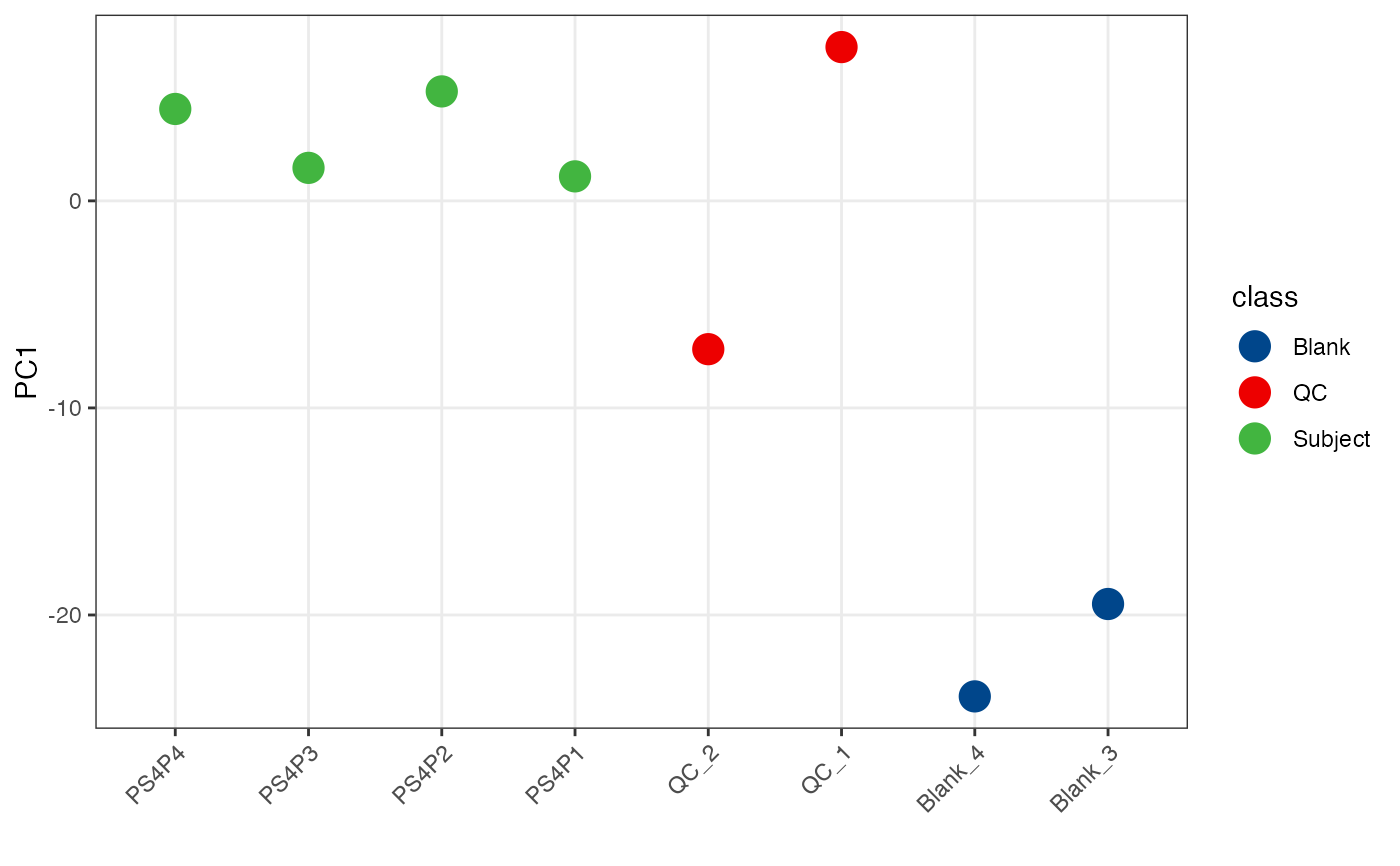Boxplot for each samples.

massqc_pca_pc1(
object,
color_by,
order_by,
point_alpha = 0.8,
point_size = 3,
desc = FALSE
)

## Arguments

object

tidymass-class object.

color_by

which column (sample_info) is used to color samples

order_by

which column (sample_info) is used to order samples

point_alpha

point_alpha

point_size

point_size

desc

desc

ggplot2 plot.

## Author

Xiaotao Shen shenxt1990@163.com

## Examples

library(massdataset)
library(ggplot2)
data("expression_data")
data("sample_info")
data("variable_info")

object =
create_mass_dataset(
expression_data = expression_data,
sample_info = sample_info,
variable_info = variable_info
)

object %>%
massqc_pca_pc1()
#> Warning: MVs in you object,
#>
#> will remove variables > 50% and imputate with zero.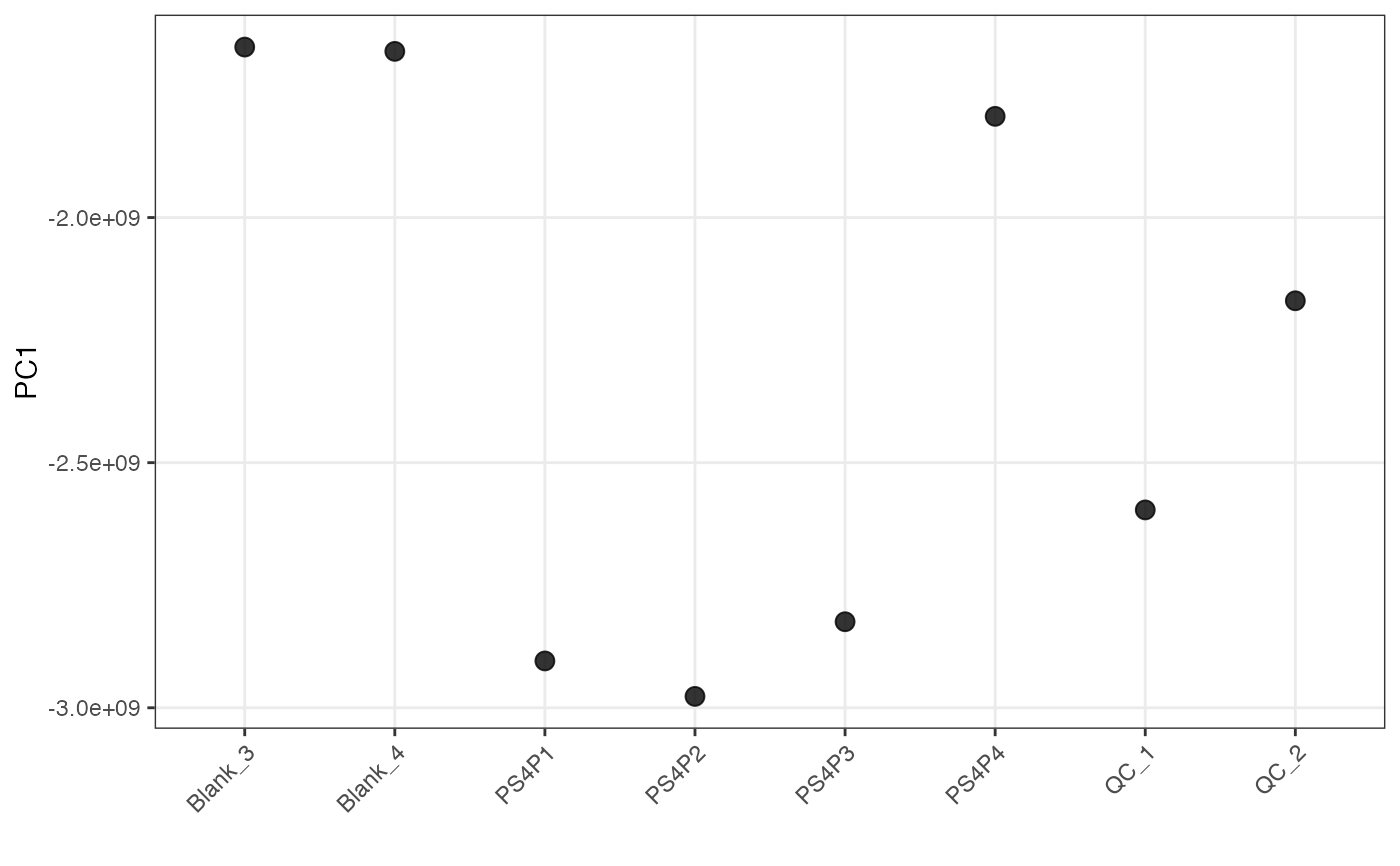object %>%
massqc_pca_pc1(color_by = "class")
#> Warning: MVs in you object,
#>
#> will remove variables > 50% and imputate with zero.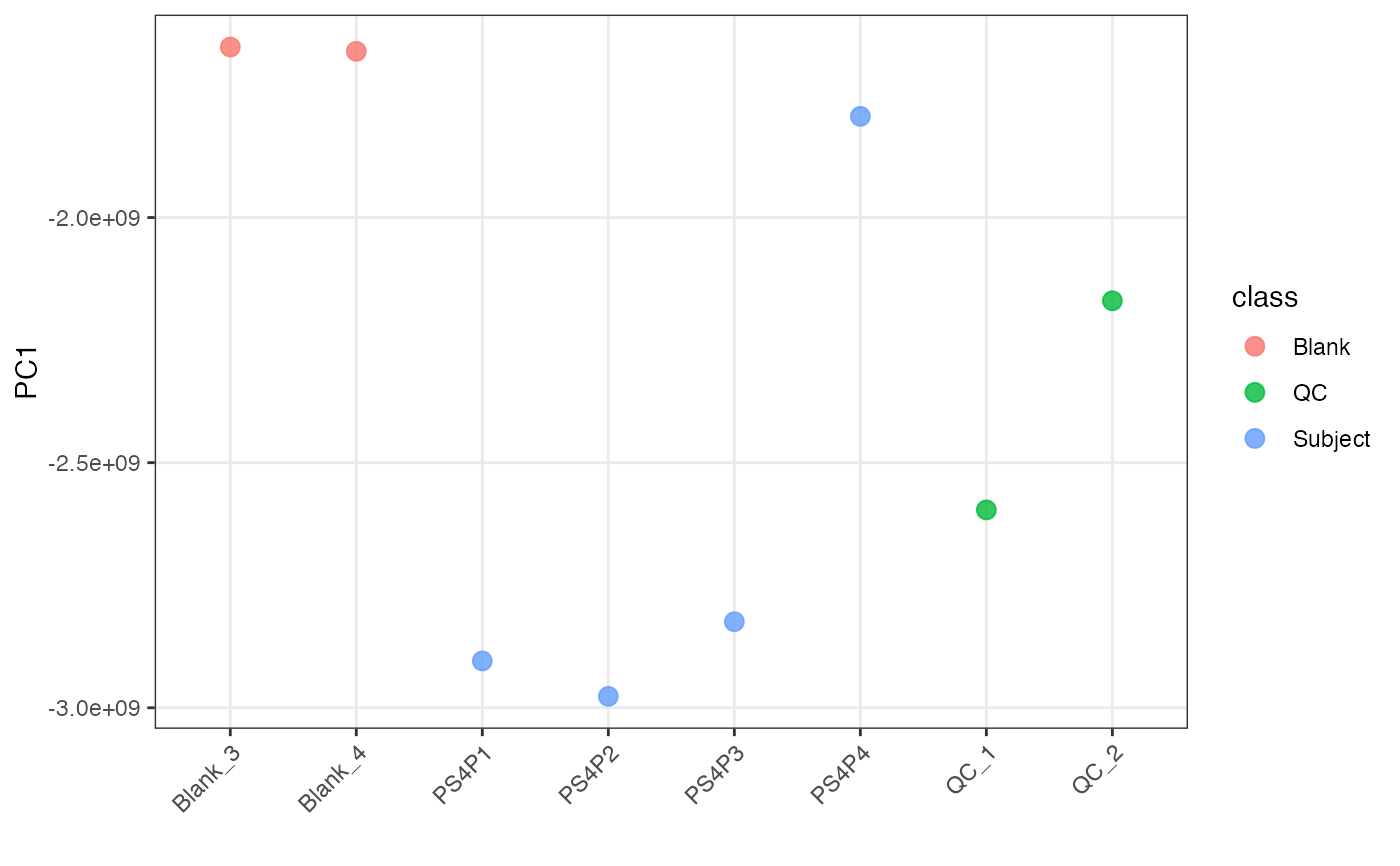object %>%
scale %>%
massqc_pca_pc1(color_by = "class")
#> Warning: MVs in you object,
#>
#> will remove variables > 50% and imputate with zero.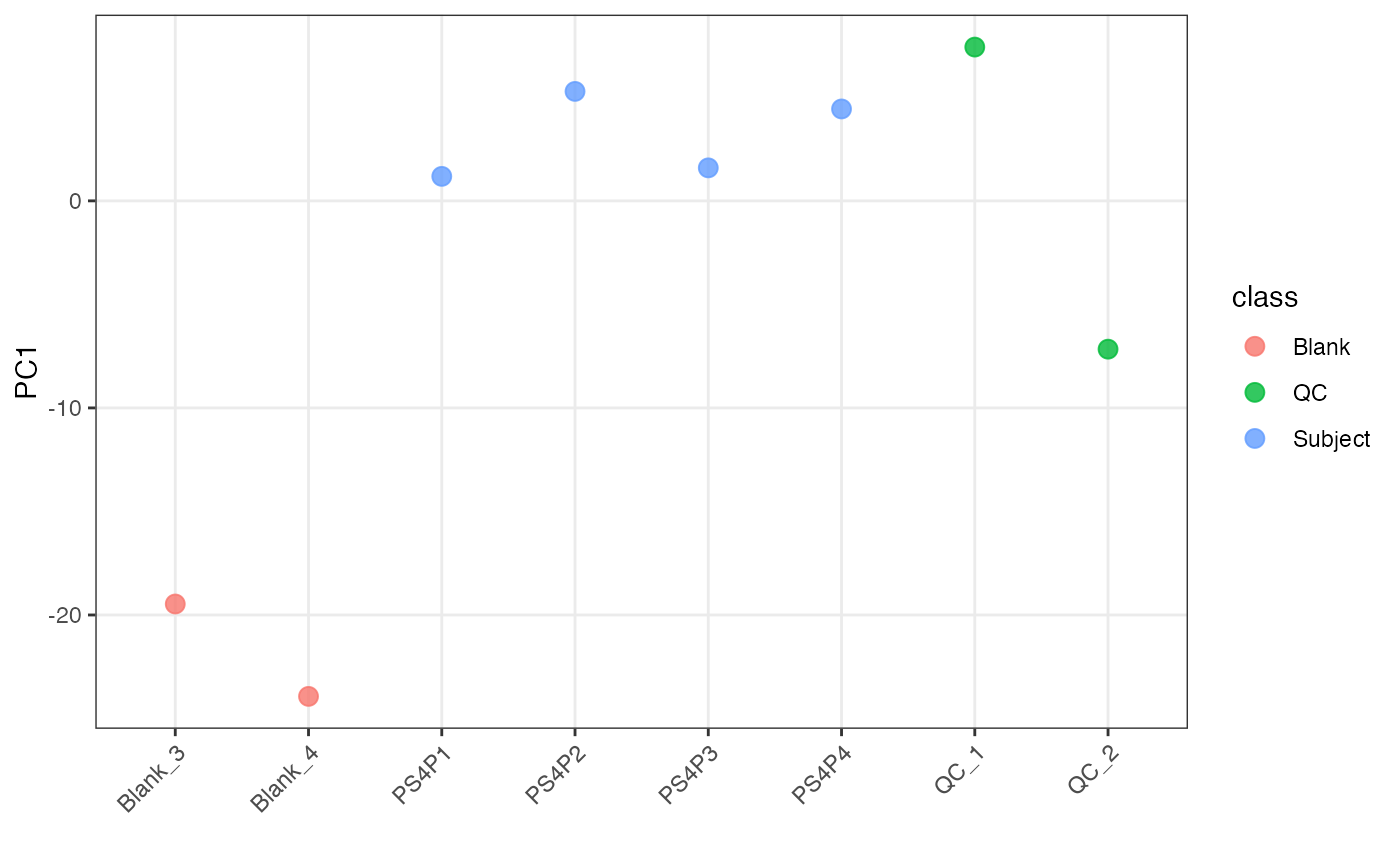object %>%
scale %>%
massqc_pca_pc1(
color_by = "class",
order_by = "injection.order",
point_alpha = 1,
point_size = 5
) +
ggsci::scale_color_lancet()
#> Warning: MVs in you object,
#>
#> will remove variables > 50% and imputate with zero.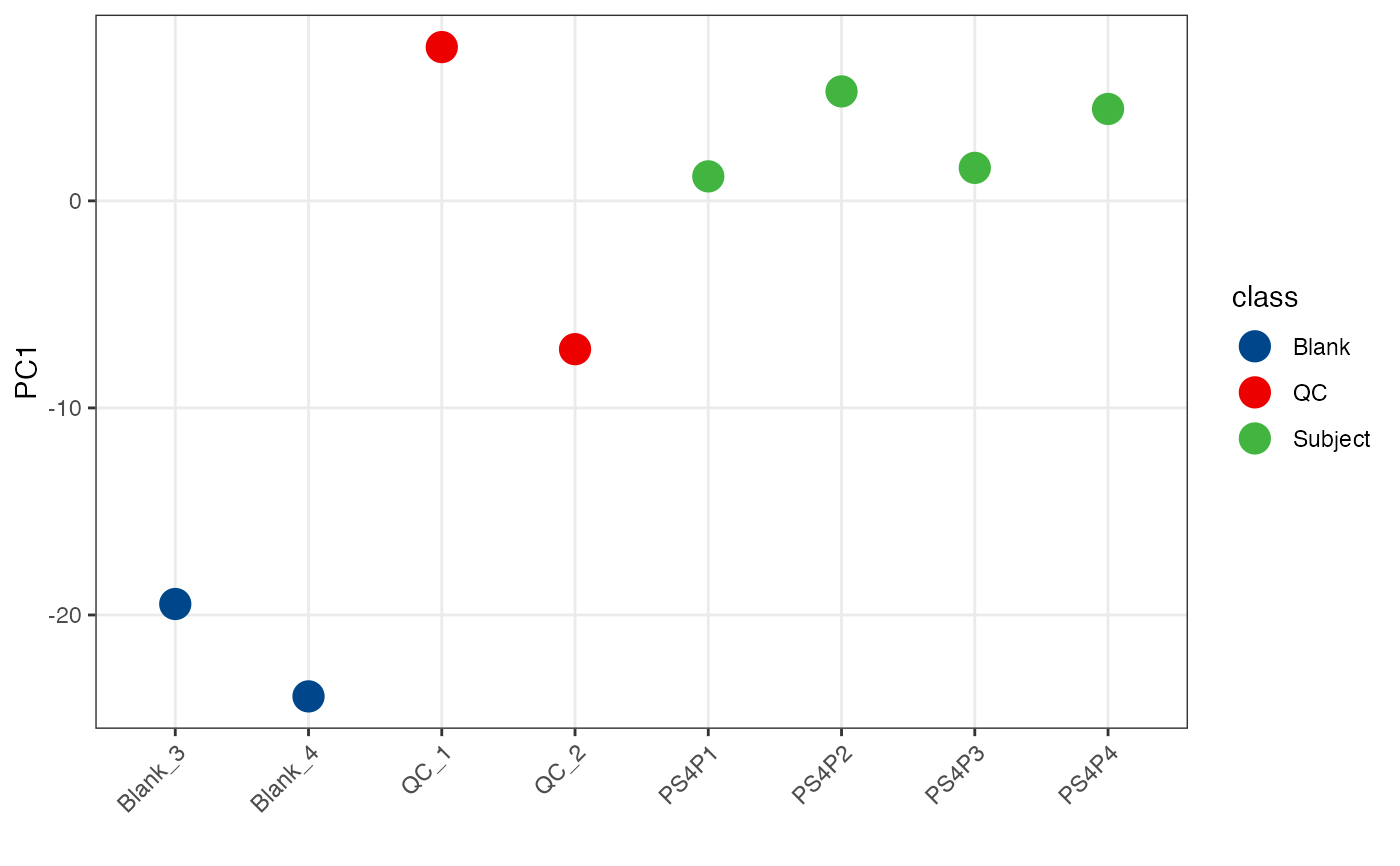object %>%
scale %>%
massqc_pca_pc1(
color_by = "class",
order_by = "injection.order",
point_alpha = 1,
point_size = 5,
desc = TRUE
) +
ggsci::scale_color_lancet()
#> Warning: MVs in you object,
#>
#> will remove variables > 50% and imputate with zero.